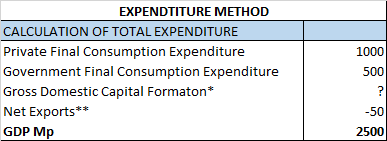Chapter 2 National Income - Part 5 Expenditure Method

Economics Class 12
Macroeconomics

#### Example 30

Calculate Gross Fixed Capital Formation from the following data:In this given

We are given GDP Mp

We have to calculate missing figure (Gross Fixed Capital formation)

Hence

Total of All expenditures =GDP Mp

Private Final Consumption Expenditure +Govt Final Consumption Expenditure +Gross Domestic Capital Formation +Net Export =GDP Mp

1000+500+Gross Domestic Capital formation -50=2500

Gross Domestic Capital formation =2500+50-500-1000

Gross Domestic Capital formation =1050

Now we know that

Gross Domestic Capital Formation = Gross Fixed Capital formation +Inventory Investment

Gross Domestic Capital Formation = Gross Fixed Capital formation +Closing Stock-Opening Stock

1050 =Gross Fixed Capital formation +200-300

1050 =Gross Fixed Capital formation -100

1050+100 =Gross Fixed Capital formation

Gross Fixed Capital formation =1050+100=1150

Learn in your speed, with individual attention - Teachoo Maths 1-on-1 Class